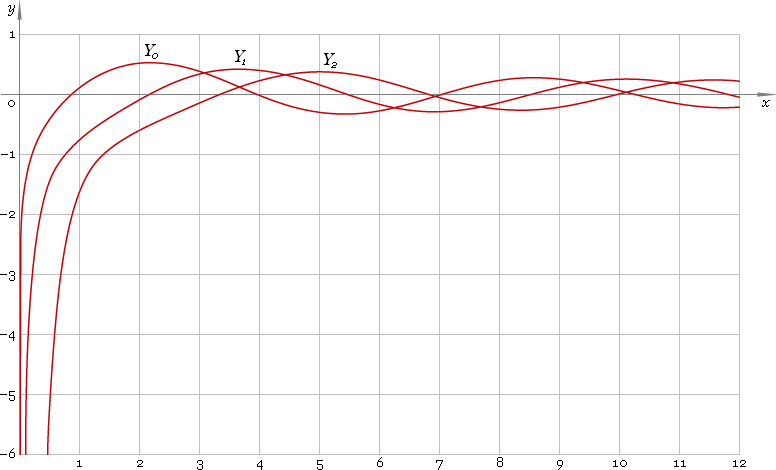The Art of Mathematics

# Y — Bessel function of the second kind

## 1. Definition

By definition Bessel function is solution of the Bessel equation

z2 w′′ + z w′ + (z2 − ν2) w = 0

As second order equation it has two solutions, second of which has singularity at 0 and is called Bessel function of the second kind — Yν. Parameter ν is called order of the function.

First solution has no singularity at 0 and is called Bessel function of the first kind — Jν.

## 2. Graph

Bessel functions of the second kind defined everywhere on the real axis, at 0 functions have singularity, so their domain is (−∞, 0)∪(0, +∞). Graphs of the first three representatives of the second kind Bessel function family depicted below — fig. 1.Fig. 1. Graphs of the Bessel functions of the second kind y = Y0(x), y = Y1(x) and y = Y2(x).

## 3. Identities

Next order recurrence:

Yν+1(z) = 2ν /z Yν(z) − Yν−1(z)

Negative argument:

Yν(−z) = eiπν Yν(z) + i 2 cos(πν) Jν(z) = cos(πν) Yν(z) + i [2 cos(πν) Jν(z) − sin(πν) Yν(z)]

For the case of integer order ν=n the negative argument identity can be simplified down to:

Yn(−z) = (−1)n Yn(z) + i (−1)n 2 Jn(z)

and for the case of half-integer order ν=n+1/2 the identity can be simplified down to:

Yn+1/2(−z) = i (−1)n+1 Yn+1/2(z)

Reflection — negative order:

Y−ν(z) = cos(πν) Yν(z) + sin(πν) Jν(z)

For the case of integer order ν=n the reflection identity can be simplified down to:

Y−n(z) = (−1)n Yn(z)

and for the case of half-integer order ν=n+1/2 the identity can be simplified down to:

Y−n−1/2(z) = (−1)n Jn+1/2(z)

## 4. Derivative

Bessel function of the second kind derivative:

Y′ν(z) = Yν−1(z) − ν Yν(z) /z Y′ν(z) = ν Yν(z) /z − Yν+1(z) Y′ν(z) = [Yν−1(z) − Yν+1(z)] /2

In particular:

Y′0(z) = −Y1(z)

## 5. Interface

Bessel function call looks like

``Y(ν, z);``

where ν is the function real order, and z — function complex argument.

## 6. How to use

To calculate Bessel function of the second kind of the 0 order of the number:

``Y(0, 1.5+i);``

To get Bessel function of the second kind of the 1.2 order of the current result:

``Y(1.2, rslt);``

To get Bessel function of the second kind of the 2.5 order of the number z in calculator memory:

``Y(2.5, mem[z]);``

## 7. Support

Bessel function of the second kind of real order and complex argument is supported in professional version of the Librow calculator.